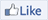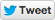22 April 2014Article: A million dollars for an imaginary game of poolThe things mathematicians imagine are often perfect. A mathematical sphere is smoother than any ball we could ever make, because it is made of ideas, not atoms. But mathematicians don’t just imagine simple shapes. This year’s winner of the million-dollar Abel prize is famous for imagining a mathematical pool table!

A pool table is designed for rolling balls on. There is a bouncy cushion around the outside to keep the balls on the table and several pockets spaced around the cushion. The aim is to hit balls into each other and bounce them into the pockets.

Yakov Sinai’s mathematical pool tables are a bit different. There are no pockets, and there is only one ball. And since it’s only the idea of a table, it’s perfect – the cushions are smooth and perfectly bouncy, and the ball never slows down. Instead it keeps bouncing around the table forever.

Yakov found that a rectangular pool table was a bit boring. The ball kept bouncing around at the same angles, so it was easy to understand where it was going to go. After looking at different shapes for his pool tables, Yakov settled on a square table with a big circular cushion in the middle.

This new table was much more interesting than rectangular ones. Whenever the ball ran into the circular cushion, it bounced off at a different angle. Yakov could predict each angle as it happened, but over a long period of time the ball moved almost randomly. Yakov eventually proved that no matter where you started the ball, it would eventually visit every part of the table, moving in every possible direction.

Yakov’s pool table is more than an imaginary game – physicists use pool tables to describe how gases work. Yakov’s work, including his pool table, is also very important in probability and statistics. Yakov won the Abel prize because of all his work, not just his pool tables. But maybe there are mathematical secrets lurking in other games too!

Yakov is a professor at Princeton University, read what they have to say about the prize
More coverage from Nature
Play MacBilliards, and learn about some unsolved questions on triangular pool tablesTry this: Pool trick shotsPut an obstacle between the two balls.Aim for a point on the cushion halfway between the two balls.Line up two balls with a pocket, then roll the white ball into them.Combine the two tricks!A much harder trick – when you hit the white ball into the middle of this shape, the six coloured balls should each go into a different pocket!NOTE: If you don’t know how to use a pool cue to hit balls, ask someone to teach you. It’s not hard, but if you do it incorrectly you might damage the table.

### You will need

• A pool table
• Three pool balls – we used a white, a yellow and a red ball
• A cue stick

### Off the cushion

1. Put the white ball in the centre of the table.
2. Imagine a line running straight down the middle of the table, long ways. Put a different ball somewhere along that line.
3. Put an obstacle such as a pool triangle in between the two balls. Your aim is to hit the white ball into the other ball.
4. To work out where to aim the ball, imagine a line between the white ball and the target. Divide that line in half, and then trace a line at right angles from your imaginary point out to the cushion. If you aim at that point on the cushion, you should make the shot.
5. Pool shots can be tricky, so don’t give up if you miss the first few times!

### Bouncing balls

1. Put a red ball on the table. Then, put a yellow ball touching the red, so they line up with one of the pockets. Make sure the yellow ball is closer to the pocket than the red ball is.
2. Take the white ball and roll it into the red ball. Where does the yellow ball roll to?
3. Set it up again, and roll the white ball in from a different angle. What do you notice?

### Putting it together

1. Put the white ball in the centre of the table.
2. Imagine a line down the centre of the table. Put the red ball on that line, and then put the yellow ball touching the first, so they line up with a pocket.
3. Put an obstacle in between the white ball and the target balls.
4. See if you can put the yellow ball into the pocket!

### What’s happening?

Pool is a game of angles. A good player can predict where each ball will be after several collisions. However, it takes a lot of practice to be that good, and it takes a lot of skill to hit the ball exactly the way you want.

When a ball hits a cushion, the angle it comes in at is the same size as the angle it comes out at. If the ball hits at a 45° angle, it will come out at the same angle. If it just grazes the cushion, it’ll stay close.

When two balls hit, they push against each other. The direction they push depends on where the two balls collide, not what direction the balls are moving in. This is why it can be easier to sink the ball in the second part of this activity – the back ball hits the front ball at the right spot to push it towards the pocket.

This activity might take a few attempts, but don’t give up! With some practice, you can master these tricks and maybe even come up with some of your own.

Play an online pool gameBrainteaser questionThere are 15 balls in a pool set, numbered 1 to 15. What is the sum of all those numbers?Websites### See maths!

Maths is really interesting, but it can also be beautiful.

### Do maths!

Martin Gardiner had a very mathematical way of making as much gold as you like!

Did you know? Pool isn’t the only game that mathematicians have studied. Blaise Pascal and Pierre de Fermat worked out the foundations of probability theory to help a gambler with a dice game!

 Brainteaser answer1 + 2 + … + 15 = 120 A quick way to work this out is to pair up the largest number with the smallest, the second largest with the second smallest, and so on. Then you get: (15 + 1) + (14 + 2) + … + 8 Each pair adds up to 16, and there are seven pairs, so we get: 16 + 16 +… + 8 7 x 16 + 8 = 120

Our partners Maths and Stats by Email is published by CSIRO. The Australian Bureau of Statistics and the Australian Mathematical Sciences Institute are proud partners of Maths and Stats by Email. The mission of the Australian Bureau of Statistics is to assist and encourage informed decision making, research and discussion within governments and the community, by leading a high quality, objective and responsive national statistical service. The Australian Mathematical Sciences Institute's mission is the radical improvement of mathematical sciences capacity and capability in the Australian community through the support of high quality mathematics education for all young Australians.